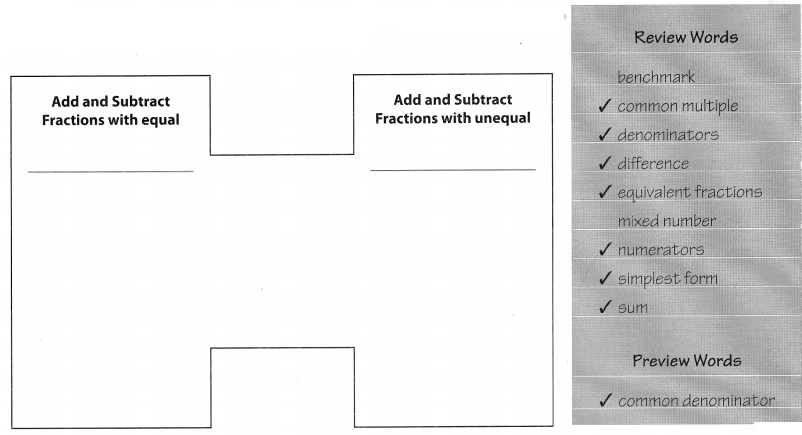# Texas Go Math Grade 5 Unit 2 Answer Key Number and Operations: Fractions

Refer to our Texas Go Math Grade 5 Answer Key Pdf to score good marks in the exams. Test yourself by practicing the problems from Texas Go Math Grade 5 Unit 2 Answer Key Number and Operations: Fractions.

## Texas Go Math Grade 5 Unit 2 Answer Key Number and Operations: Fractions

Show What You Know

Check your understanding of important skills.

Part of a Whole: Write a fraction to name the shaded part.

Question 1.number of total parts ___________
fraction ___________
The above figure is a hexagon. It is divided into six parts.
number of shaded parts are 5.
number of total parts is 6
fraction 5/6

Question 2.number of total parts ___________
fraction ___________
The shape of the above figure is a circle.
number of shaded parts are 1
number of total parts is 4
fraction 1/4

Write the sum or difference In simplest form.

Question 3.
$$\frac{3}{6}$$ + $$\frac{1}{6}$$ = ___________
$$\frac{3}{6}$$ + $$\frac{1}{6}$$
The denominator of both the fraction is same.
We can add the numerator directly.
$$\frac{3}{6}$$ + $$\frac{1}{6}$$ = $$\frac{4}{6}$$

Question 4.
$$\frac{4}{10}$$ + $$\frac{1}{10}$$ = ____________
$$\frac{4}{10}$$ + $$\frac{1}{10}$$
The denominator of both the fraction is same.
We can add the numerator directly.
$$\frac{4}{10}$$ + $$\frac{1}{10}$$ = (4 + 1)/10 = $$\frac{5}{10}$$

Question 5.
$$\frac{7}{8}$$ – $$\frac{3}{8}$$ = _____________
$$\frac{7}{8}$$ – $$\frac{3}{8}$$
The denominator of both the fraction is same.
We can subtract the numerator directly.
$$\frac{7}{8}$$ – $$\frac{3}{8}$$ = (7 – 3)/8 = $$\frac{4}{8}$$

Question 6.
$$\frac{9}{12}$$ – $$\frac{2}{12}$$ = _____________
Given the fractions,
$$\frac{9}{12}$$ – $$\frac{2}{12}$$
The denominator of both the fraction is same.
We can subtract the numerator directly.
$$\frac{9}{12}$$ – $$\frac{2}{12}$$ = (9-2)/12 = $$\frac{7}{12}$$

Equivalent Fractions
Write an equivalent fractions.

Question 7.
$$\frac{3}{4}$$ _________
Equivalent fractions are fractions with different numbers representing the same part of a whole.
$$\frac{3}{4}$$ × $$\frac{2}{2}$$ = $$\frac{6}{8}$$

Question 8.
$$\frac{9}{15}$$ __________
Equivalent fractions are fractions with different numbers representing the same part of a whole.
$$\frac{9}{15}$$ × $$\frac{2}{2}$$ = $$\frac{18}{30}$$

Question 9.
$$\frac{24}{40}$$ ____________
Equivalent fractions are fractions with different numbers representing the same part of a whole.
$$\frac{24}{40}$$ × $$\frac{2}{2}$$ = $$\frac{48}{80}$$

Question 10.
$$\frac{5}{7}$$ ____________
Equivalent fractions are fractions with different numbers representing the same part of a whole.
$$\frac{5}{7}$$ × $$\frac{2}{2}$$ = $$\frac{10}{14}$$

Vocabulary Builder

Visualize It

Use the ✓ words to complete the H-diagram.Understand Vocabulary

Draw a line to match the word with its definition.Reading To get the right answer to a mathematics problem, you need to make sure you understand the question.

Problem 1.
Three friends ordered a pizza with 8 slices. Jeanette ate of the pizza. Marissa ate of the pizza. Ariel ate the rest. How many slices of Pizza did Ariel eat?
A. 1 slice
B. 2 slices
C. 4 slices
D. 6 slices

Thinking Through the Problem

Understand the question You want to know how many slices Ariel ate. Will your answer be a fraction or whole number?

Plan Find out what fraction of the pizza Ariel ate. Look at the numerator to tell how many pizza slices Ariel ate.

Writing Now it’s your turn. Answer Problem 2. Then write about how you solved the problem, step by step.:

Problem 2.
The Perez family ordered a large pizza for dinner, A large pizza is divided into 8 slices. Marco ate $$\frac{3}{8}$$ of the pizza. Ramon ate 1 slice more than Marco. Emilio ate the rest. How much of the pizza did Emilio eat?A. $$\frac{1}{8}$$ of the pizza
B. $$\frac{2}{8}$$ of the pizza
C. $$\frac{4}{8}$$ of the pizza
D. $$\frac{5}{8}$$ of the pizza
Given,
The Perez family ordered a large pizza for dinner, A large pizza is divided into 8 slices.
Marco ate $$\frac{3}{8}$$ of the pizza.
Ramon ate 1 slice more than Marco. Emilio ate the rest.
$$\frac{3}{8}$$ + $$\frac{1}{8}$$ = $$\frac{4}{8}$$
$$\frac{4}{8}$$ + $$\frac{4}{8}$$ = 1
Thus the correct answer is option C.

Action Fractions
Object of the Game Practice comparing fractions

Materials
Number/Symbol Cards: 2 sets labeled 1, 2, 3, 4, 6, 8
Number of Players 2

Set Up
Give each player 2 sets of number cards. Players shuffle their cards and place them face down in a stack.

How to Play

(1) One player shuffles arid deals all cards facedown. Players stack their cards.

(2) Players take 3 cards from the top of their stacks. Using 2 of the 3 cards, each player makes a fraction whose numerator is less than or equal to its denominator. The unused card is returned to the bottom of the player’s stack.(3) Players compare the fractions. The player with the greater fraction earns 1 point. If the fractions are equivalent, each player earns 1 point.

(4) Repeat Steps 2 and 3. The player with more points after all the cards have been used is the winner.